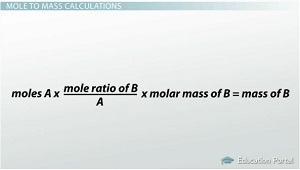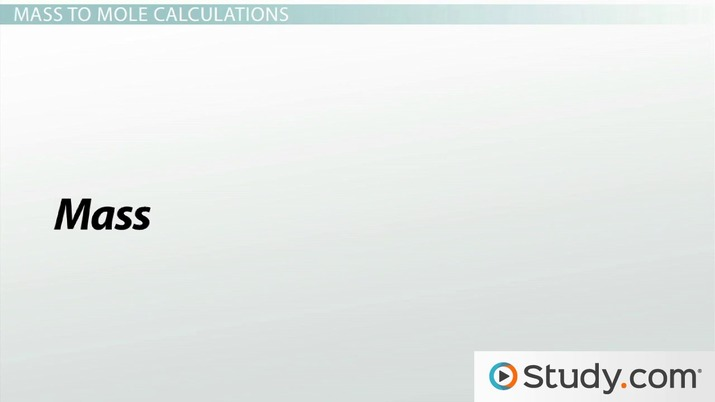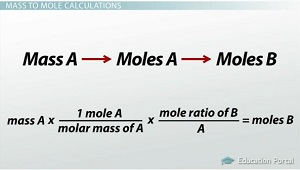# Mass-to-Mass Stoichiometric Calculations

Lesson Transcript
Instructor: Amy Meyers

Amy holds a Master of Science. She has taught science at the high school and college levels.

Moles and mass are commonly used to express the amount of substances in stoichiometric calculations. Learn how to use equations and ratios to calculate moles to mass, moles to moles, mass to moles, or mass to mass in stoichiometric calculations. Updated: 11/17/2021

## Mole to Mass Calculations

You have previously learned about mole ratios and how to make mole to mole calculations from balanced chemical equations. This lesson will teach you how to relate the mole ratio to the amounts of substances in grams. For either reactants or products, you will learn how to determine mass when given moles, moles when given mass and mass when given mass.

To start, you know if you have an equation such as 2H2 + O2 → 2 H2 O, your mole ratios are:

• 2 moles H2 per 1 mole O2
• 2 moles H2 per 2 moles H2 O
• 1 mole O2 per 2 moles H2 O

If you want to convert moles to mass, you have to do the following conversions. First, convert moles of substance A into moles of substance B. Then, convert moles of B into mass of B. Moles A → moles B → mass B.The main equation is: moles A x (mole ratio of B/A) x molar mass of B = mass of B. The way you would use this in an actual problem is the following. You are asked how many grams of glucose are produced when 3 moles of water react with carbon dioxide. Start with your balanced equation: 6 CO2 + 6 H2 O → C6 H12 O6 + 6 O2.

Let water equal A, and let glucose equal B. From the balanced reaction, you know the mole ratio of B/A is (1 mole C6 H12 O6 )/(6 moles H2 O). So, in the original equation, you have both of these terms. Moles A x (mole ratio of B/A) x molar mass of B = mass of B.

• Moles A = 3 moles H2 O
• Mole ratio of B/A = (1 mole C6 H12 O6 )/(6 moles H2 O)

You have learned previously how to determine the amount of grams in one mole of a substance. For this problem, I will just tell you it is 180g glucose per 1 mole glucose. Now you have all three pieces of data you need to figure out the solution.

• Moles A x (mole ratio of B/A) x molar mass of B = mass of B
• (3 moles H2 O)/1 x (1 mole C6 H12 O6 )/(6 moles H2 O) x (180g C6 H12 O6 )/(1 mole C6 H12 O6 ) = grams of C6 H12 O6

The 'moles H2 O' and 'mole C6 H12 O6 ' cross out: (3/1) x (1/6) x (180g/1) = grams of C6 H12 O6 .

90g = grams of C6 H12 O6!An error occurred trying to load this video.

Try refreshing the page, or contact customer support.

Coming up next: Stoichiometry: Calculating Relative Quantities in a Gas or Solution

### You're on a roll. Keep up the good work!

Replay
Your next lesson will play in 10 seconds
• 0:08 Mole to Mass Calculations
• 3:24 Mass to Mole Calculations
• 5:07 Mass to Mass Calculations
• 6:29 Lesson Summary
Save Save

Want to watch this again later?

Timeline
Autoplay
Autoplay
Speed Speed

## Mass to Mole Calculations

Let's try another example, this time converting mass to moles. The conversions you need to make are:

• Mass A → moles A → moles B
• Mass A x (1 mole A)/(molar mass of A) x (mole ratio of B)/A = moles B

Start with the balanced equation of 5C + 2 SO2 → CS2 + 4CO. If 8g of SO2 how many moles of CS2 are formed?To unlock this lesson you must be a Study.com Member.

### Register to view this lesson

Are you a student or a teacher?